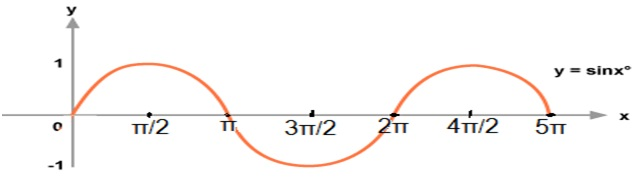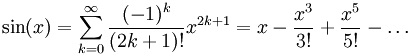Q:

# C program for sin(x) series## Sin x Series:## Program for sin(x) series in C

``````#include <stdio.h>
#include <math.h>

int fac(int x)
{
int i,fac=1;
for(i=1;i<=x;i++)
fac=fac*i;
return fac;
}

int main()
{
float x,Q,sum=0;
int i,j,limit;

printf("Enter the value of x of sinx series: ");
scanf("%f",&x);

printf("Enter the limit upto which you want to expand the series: ");
scanf("%d",&limit);

Q=x;
x = x*(3.1415/180);

for(i=1,j=1;i<=limit;i++,j=j+2)
{
if(i%2!=0)
{
sum=sum+pow(x,j)/fac(j);
}
else
sum=sum-pow(x,j)/fac(j);
}

printf("Sin(%0.1f): %f",Q,sum);
return 0;
}``````

Output

```Enter the value of x of sinx series: 40
Enter the limit upto which you want to expand the series: 5
Sin(40.0): 0.642772```